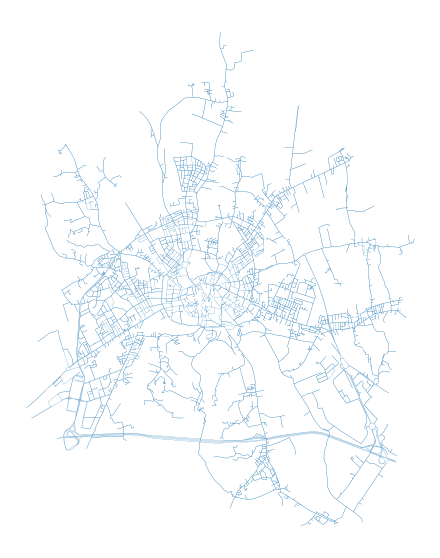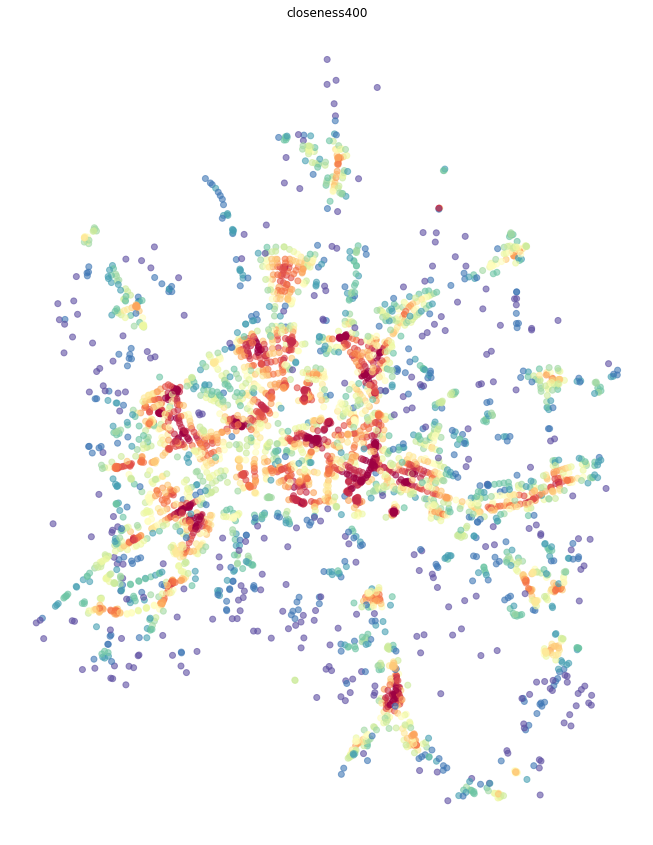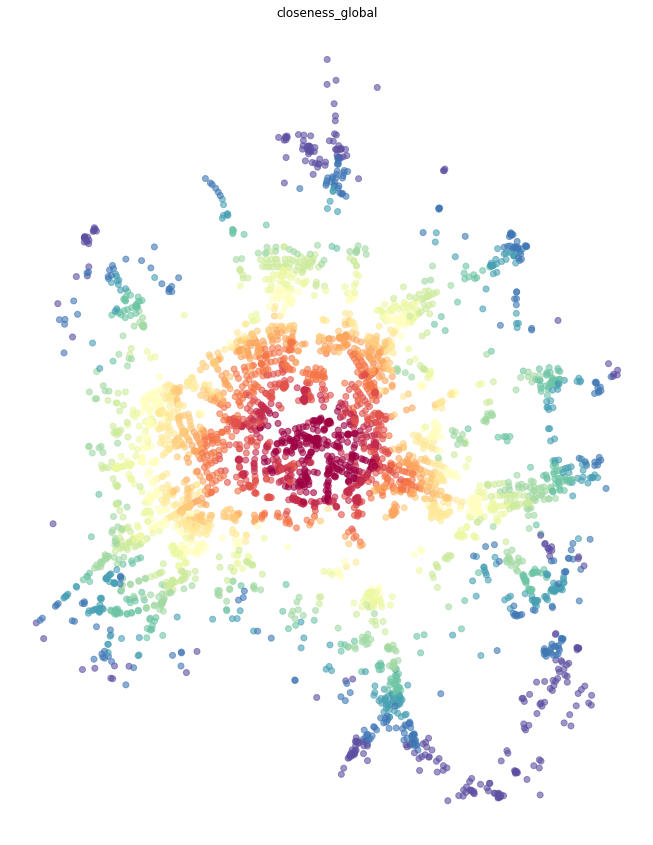Multiple Centrality Assessment (MCA) is an approach to street network analysis developed by Porta and Latora (2006). It's main aim is to understand the structure of street network of our cities from the perspective of the importance and position of each street/intersection within the whole network as expressed by various centralities. Momepy can do all types of MCA-based centrality analysis as were developed through the years.

The aim of this notebook is to illustrate how to measure different centralities using momepy. For the theoretical background, please refer to the work of Porta et al.

In :
import geopandas as gpd
import momepy
import osmnx as ox
import matplotlib.pyplot as plt


In the ideal case, momepy expects LineString GeoDataFrame containing street network as a starting point. Either we have our own, or we can use osmnx to dowload network from OSM. In this notebook, we will look at Vicenza, Italy.

In :
streets_graph = ox.graph_from_place('Vicenza, Italy', network_type='drive')
streets_graph = ox.project_graph(streets_graph)


Code above dowloaded network from OSM and projected it. At this point, streets_graph is networkX Graph object, similar to the one we will use in momepy. In theory, you can use it directly. However, momepy when converting GeoDataFrame to network ensures that all attributes are set and compatible with morphometric functions, so we do recommend saving graph to gdf and let momepy do the conversion back to graph.

In :
edges = ox.save_load.graph_to_gdfs(streets_graph, nodes=False, edges=True,
node_geometry=False, fill_edge_geometry=True)

In :
f, ax = plt.subplots(figsize=(10, 10))
edges.plot(ax=ax, linewidth=0.2)
ax.set_axis_off()
plt.show()To measure centrality, we have to convert gdf to graph. For that, we can choose from two options how to represent street network within graph. First and the most straightforward one is the primal approach (Porta et al., 2006) where street is represented by graph edge and intersection by node.

## Primal graph¶

We can generate networkX.MultiGraph, which is used within momepy, using gdf_to_nx.

In :
primal = momepy.gdf_to_nx(edges, approach='primal')


### Closeness centrality¶

Closeness centrality could be simplified as average distance to every other node from each node. As such, it can be measured on the whole network (Global Closeness Centrality) or wihtin certain reach only (Local Closeness Centrality).

#### Local closeness¶

To measure local_closeness_centrality we need to specify radius (how far we should go from each node). We can use topological distance (e.g. 5 steps, then radius=5) or metric distance (e.g. 400 metres) - then radius=400 and distance= lenght of each segment saved as a parameter of each edge. By default, momepy saves length as mm_len.

Weight parameter is used for centrality calculation. Again, we can use metric weight (using the same attribute as above) or no weight (weight=None) at all. Or any other attribute we wish.

In :
primal = momepy.local_closeness_centrality(primal, radius=400, name='closeness400', distance='mm_len', weight='mm_len')

100%|██████████| 4075/4075 [00:09<00:00, 451.13it/s]

In :
nodes = momepy.nx_to_gdf(primal, lines=False)
f, ax = plt.subplots(figsize=(15, 15))
nodes.plot(ax=ax, column='closeness400', cmap='Spectral_r', scheme='quantiles', k=15, alpha=0.6)
ax.set_axis_off()
ax.set_title('closeness400')
plt.show()#### Global closeness¶

Global closeness centrality is a bit simpler as we do not have to specify radius and distance, the rest remains the same.

In :
primal = momepy.closeness_centrality(primal, name='closeness_global', weight='mm_len')


Note: Computing global centrality on larger network can take a while to compute, be patient.

In :
nodes = momepy.nx_to_gdf(primal, lines=False)
f, ax = plt.subplots(figsize=(15, 15))
nodes.plot(ax=ax, column='closeness_global', cmap='Spectral_r', scheme='quantiles', k=15, alpha=0.6)
ax.set_axis_off()
ax.set_title('closeness_global')
plt.show()### Betweenness¶

Betweenness centrality measures the importance of each node or edge for the travelling along the network. It measures how many times is each node/edge used if we walk using the shortest paths from each node to every other.

We have two options how to measure betweenness on primal graph - on nodes or on edges.

#### Node-based¶

Node-based betweenness, as name suggests, measures betweennes of each node - how many times we would walk through node.

In :
primal = momepy.betweenness_centrality(primal, name='betweenness_metric_n', mode='nodes', weight='mm_len')

In :
nodes = momepy.nx_to_gdf(primal, lines=False)
f, ax = plt.subplots(figsize=(15, 15))
nodes.plot(ax=ax, column='betweenness_metric_n', cmap='Spectral_r', scheme='quantiles', k=7, alpha=0.6)
ax.set_axis_off()
ax.set_title('betweenness_metric_n')
plt.show()